# How to Calculate Percentage of Total in Excel

In this article, we will learn methods of calculating the percentage of total in Excel. Calculating percentage is basic task in any field of work. We always want to know how much work is done. In school/collage we always calculated our attendance percentage carefully to be able to appear in the exam. So calculating percentage is basic and you must know how to Calculate Percentage in Excel too.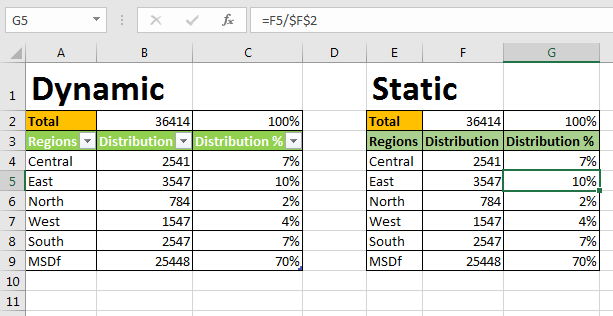Basic Percentage formula to calculate percentage is:

=(part/Total)*100

Let’s do this in excel. We don’t have any specific excel percentage formula and it is not required as per me and MS apparently ????.

#### How to Calculate Percentage of Total

Let’s say you distributed your product in five regions of country. You would like to know what percentage of total product is distributed in each region. See below image.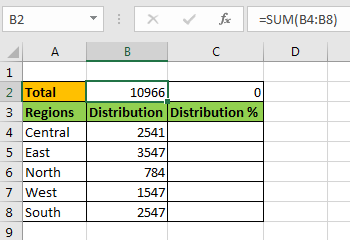I always prefer my total on the top of table, so that whenever I extend the table I do not need to replace it. And it is always visible too if rows are frozen. So yeah it has its benefits.

Now to calculate percentage of total write this Percentage formula in cell C4 and drag it down:

=B4/\$B\$2

You will see fraction numbers. Now to convert them into percentage, select the cells and press CTRL+SHIFT+(%). This is a shortcut to convert number into percentage. Same thing can be done form Number section of Home tab.
-->Goto home tab.
-->Click on % formating in Number section.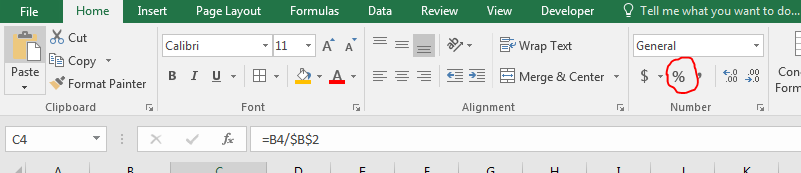And it’s done. We calculated the percentage of distribution in each region of Total distribution.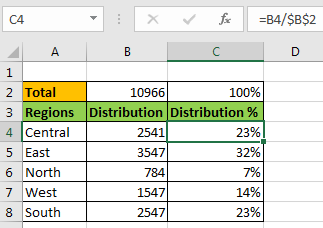Note that

• I have given absolute reference of Total and relative reference of the region. It will allow regions to change when copied in relative cell but total will not change. Learn about relative and absolute references in excel.
• In C2 we have some of the percentage, which 100%. This can be used to validate the report. It should always be 100%.

Here we use number formating to add % symbol. In the background they are actually fraction numbers. 7% mean 0.7.
If you want your percentage in whole number than use this formula instead.

=(B4/\$B\$2)*100

#### Calculate Percentage of Total without Total Cell

In above example we used a total cell. Although you would like to have that total cell but in case you don’t, write this formula to calculate percentage of total.

=B4/SUM(\$B\$4:\$B\$8)

Here we just replaced total cell with absolute function in that cell.

#### Calculate Dynamic Percentage of Total using Tables

The above examples are static. You will need to edit them if you extend your table. So how do you Calculate Percentage of Total Dynamically? We will use Tables here.

• Go to Home tab.
• Click on “Format as Table”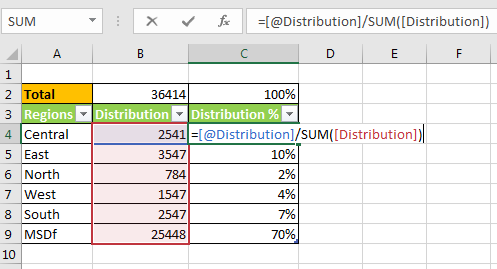Now each column is a dynamic named range. The heading are name of ranges. This makes it very easy to read and write the formulas. Write this formula in Cell C4.

=[@Distribution]/SUM([Distribution])

So yeah my friend, you can calculate % of of Total in Excel. This easy and quick. Let us know if you have any specific problem regarding calculating percentage in Excel in the comments section below.

Related Article:

How to Calculate Profit margin percentage in Excel

How to Percentage Decrease Change in Excel

How to Calculate Percentage Discount in Excel

How to Increase by percentage in Excel

Popular Articles:

50 Excel Shortcuts to Increase Your Productivity

How to use the VLOOKUP Function in Excel

How to use the COUNTIF function in Excel

How to use the SUMIF Function in Excel

Terms and Conditions of use

The applications/code on this site are distributed as is and without warranties or liability. In no event shall the owner of the copyrights, or the authors of the applications/code be liable for any loss of profit, any problems or any damage resulting from the use or evaluation of the applications/code.